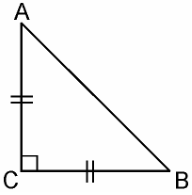# ABC is an isosceles triangle with AC = BC. If AB2 = 2AC2, prove that ABC is a right triangle.

Given that

ΔABC is an isosceles triangle having AC = BC and AB2 = 2ACIn ΔACB,

AC = BC

AB2 = 2AC2

AB2 = AC+ AC2

= AC2 + BC[Since, AC = BC]

By the Pythagoras theorem, ΔABC is a right-angle triangle.